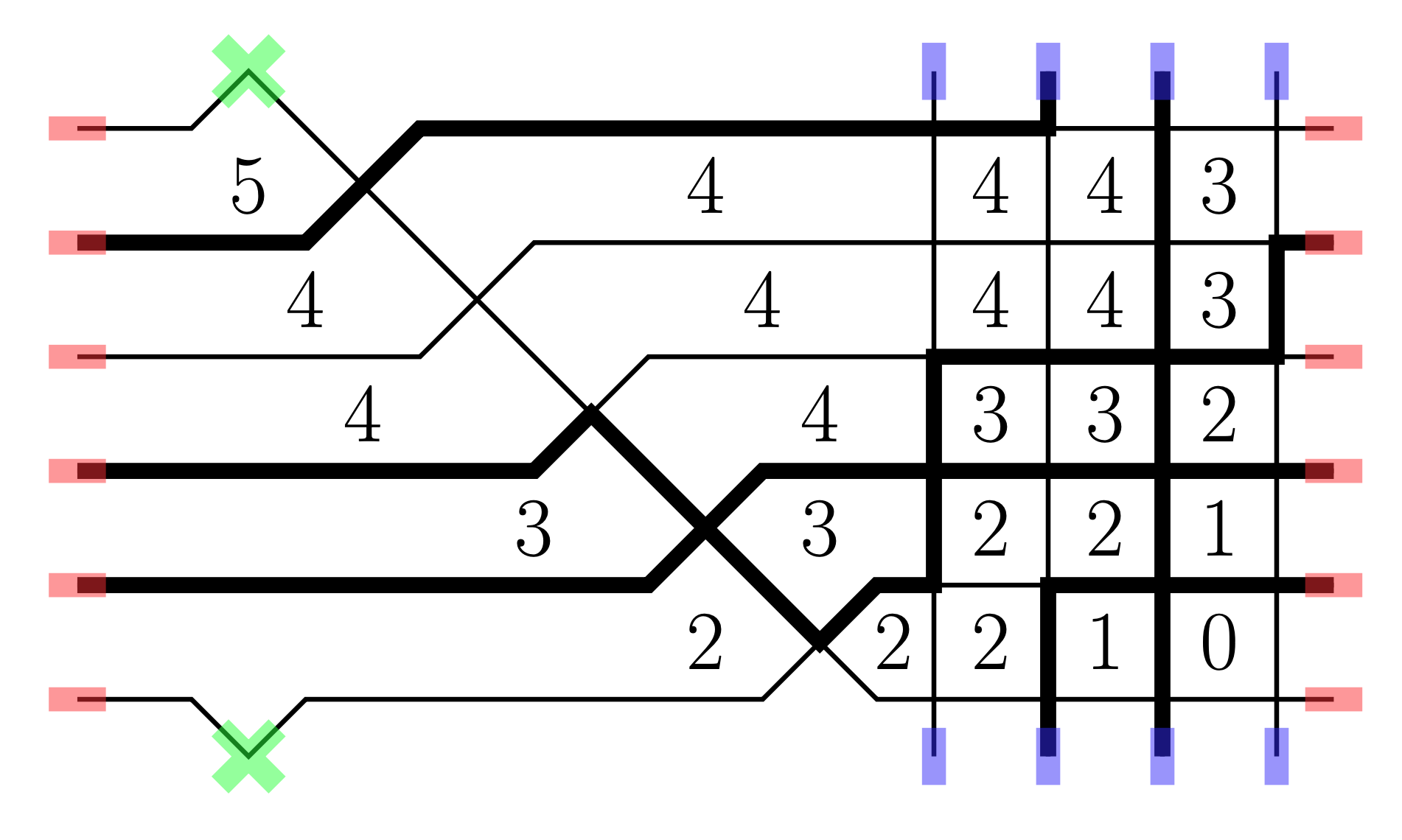# Irreversible Markov Dynamics and Hydrodynamics for KPZ States in the Stochastic Six Vertex Model

### 2022/01/28

Matthew Nicoletti, Leonid Petrov
Electron. J. Probab., to appear.arXiv:2201.12497 [math.PR]

PDF

Source

We introduce a family of Markov growth processes on discrete height functions defined on the 2-dimensional square lattice. Each height function corresponds to a configuration of the six vertex model on the infinite square lattice. We focus on the stochastic six vertex model corresponding to a particular two-parameter family of weights within the ferroelectric regime. It is believed (and partially proven, see Aggarwal, arXiv:2004.13272) that the stochastic six vertex model displays nontrivial pure (i.e., translation invariant and ergodic) Gibbs states of two types, KPZ and liquid. These phases have very different long-range correlation structure. The Markov processes we construct preserve the KPZ pure states in the full plane. We also show that the same processes put on the torus preserve arbitrary Gibbs measures for generic six vertex weights (not necessarily in the ferroelectric regime).

Our dynamics arise naturally from the Yang-Baxter equation for the six vertex model via its bijectivisation, a technique first used in Bufetov-Petrov (arXiv:1712.04584). The dynamics we construct are irreversible; in particular, the height function has a nonzero average drift. In each KPZ pure state, we explicitly compute the average drift (also known as the current) as a function of the slope. We use this to analyze the hydrodynamics of a non-stationary version of our process acting on quarter plane stochastic six vertex configurations. The fixed-time limit shapes in the quarter plane model were obtained in Borodin-Corwin-Gorin (arXiv:1407.6729).The twisted torus, a six vertex configuration, and the corresponding height function